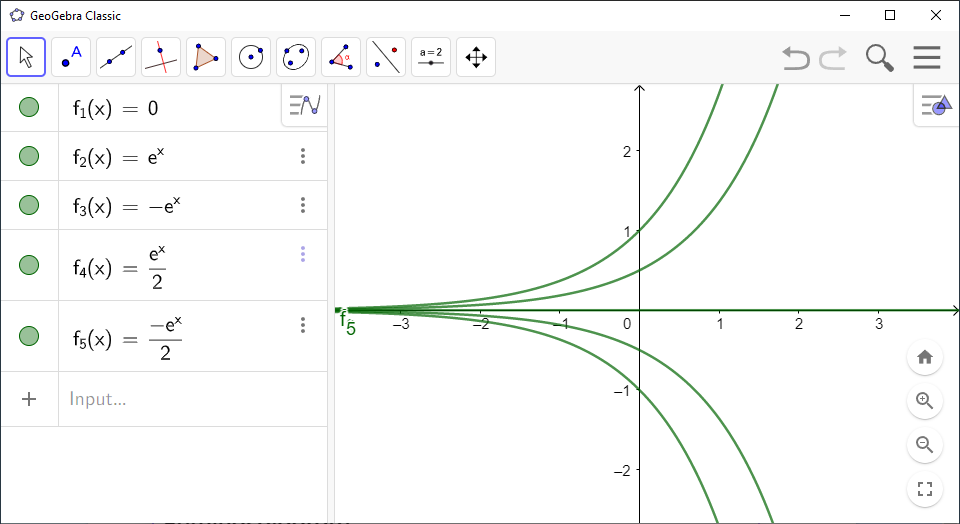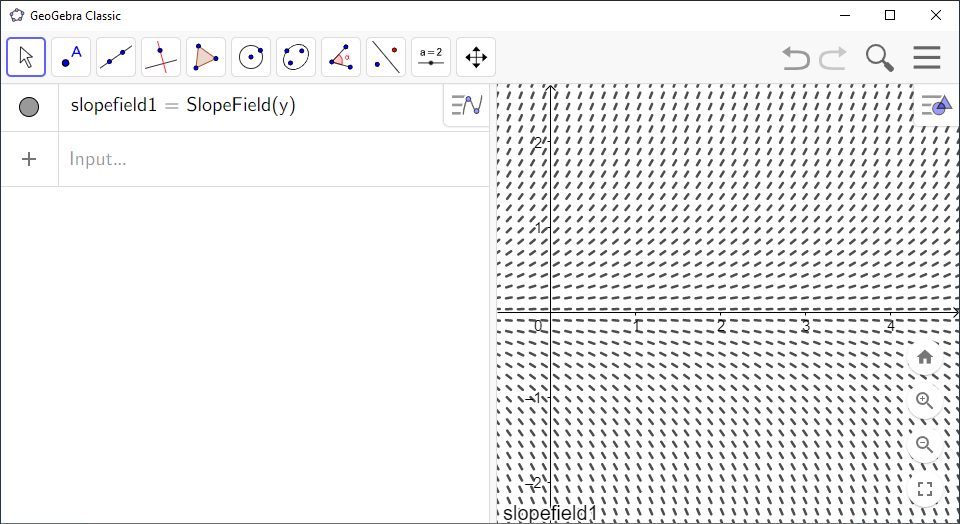# Integral Curves and Direction Fields in GeoGebra

You can use `GeoGebra` to draw integral curves and direction fields of a differential equation.

To draw integral curves in `GeoGebra`, you need to find a particular solution of the differential equation for each curve you want to draw.

`GeoGebra` Instruction 1

### IntegralCurves

1.
Open `Algebra View` and `Graphics View` under`View` in`Menu`.
2.
For each particular solution you want to draw, type

f(x) := <the particular solution>

in `Algebra View`. Press `Enter`.

3.
Do this many times with different particular solutions if you are going to make a family of curves.To draw direction fields, you have to solve the differential equation with respect to ${y}^{\prime }$ first. That is, you have to write the differential equation so that ${y}^{\prime }$ is isolated on the left-hand side of the equal sign. You do this because you need an expression for ${y}^{\prime }$.

`GeoGebra` Instruction 2

### DirectionFields

1.
Open `Algebra View` and `Graphics View` under`View` in`Menu`.
2.
Type `SlopeField(<f(x, y)>)` in `Algebra View`. Type the expression for ${y}^{\prime }$ as `<f(x,y)>`. Press `Enter`.